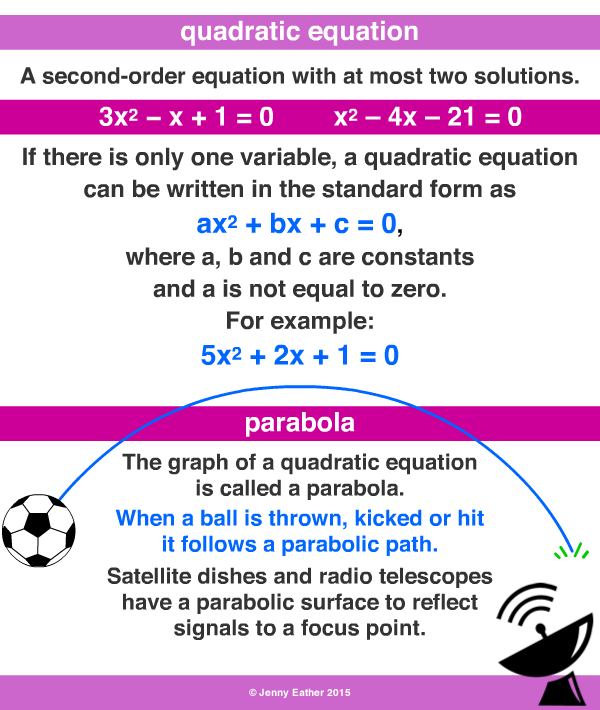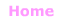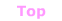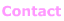Aa Bb Cc Dd Ee Ff Gg Hh Ii Jj Kk Ll Mm Nn Oo Pp Qq Rr Ss Tt Uu Vv Ww Xx Yy Zz

Qq

• an second order equation or equation of degree 2.
• the degree is equal to the largest exponent or power.
• quadratic equations contain terms with powers no higher than two.
• often in the form ax2 + bx + c = 0 where x is the variable;
a, b and c are constants and a ≠ 0.

EXAMPLES:© Jenny Eather 2014. All rights reserved.